NEET  >  Test: Molecular Orbital Theory

# Test: Molecular Orbital Theory

Test Description

## 23 Questions MCQ Test Topic-wise MCQ Tests for NEET | Test: Molecular Orbital Theory

Test: Molecular Orbital Theory for NEET 2022 is part of Topic-wise MCQ Tests for NEET preparation. The Test: Molecular Orbital Theory questions and answers have been prepared according to the NEET exam syllabus.The Test: Molecular Orbital Theory MCQs are made for NEET 2022 Exam. Find important definitions, questions, notes, meanings, examples, exercises, MCQs and online tests for Test: Molecular Orbital Theory below.
Solutions of Test: Molecular Orbital Theory questions in English are available as part of our Topic-wise MCQ Tests for NEET for NEET & Test: Molecular Orbital Theory solutions in Hindi for Topic-wise MCQ Tests for NEET course. Download more important topics, notes, lectures and mock test series for NEET Exam by signing up for free. Attempt Test: Molecular Orbital Theory | 23 questions in 23 minutes | Mock test for NEET preparation | Free important questions MCQ to study Topic-wise MCQ Tests for NEET for NEET Exam | Download free PDF with solutions
 1 Crore+ students have signed up on EduRev. Have you?
Test: Molecular Orbital Theory - Question 1

### Direction (Q. Nos. 1-14) This section contains 14 multiple choice questions. Each question has four choices (a), (b), (c) and (d), out of which ONLY ONE option is correct. Q. Assuming that Hund’s rule is violated, the bond order and magnetic nature of the diatomic molecule B2 is [IIT JEE 2010]

Detailed Solution for Test: Molecular Orbital Theory - Question 1

B2 (10 electrons)
MO electronic configuration is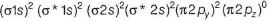Bonding electrons = 6
Anti-bonding electrons = 4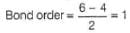No unpaired electron-diamagnetic

Test: Molecular Orbital Theory - Question 2

### In which of the following pairs of molecules/ions both the species are not likely to exist?

Detailed Solution for Test: Molecular Orbital Theory - Question 2

Species with (zero) bond order will not exist.
Electrons in orbital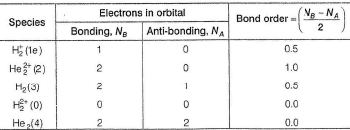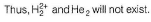Test: Molecular Orbital Theory - Question 3

### Among the following the maximum covalent character is shown by the compound

Test: Molecular Orbital Theory - Question 4

Assuming (2s-2p) mixing is not operative, the paramagnetic species among the following is

Detailed Solution for Test: Molecular Orbital Theory - Question 4

If (2s-2p) mixing is not operative, then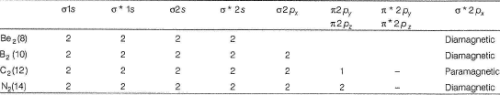Test: Molecular Orbital Theory - Question 5

Select the correct statem ent about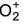and and O2.

Detailed Solution for Test: Molecular Orbital Theory - Question 5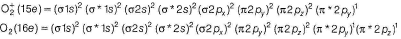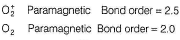Test: Molecular Orbital Theory - Question 6

The common features among the species CN-, CO, NO+ and N2 are

Detailed Solution for Test: Molecular Orbital Theory - Question 6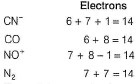Thus, all these are isoelectronic. MO electronic configuration is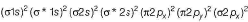No electron unpaired - diamagnetic
Bonding electrons = 10
Anti-bonding electrons = 4
Thus, bond- order =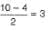Test: Molecular Orbital Theory - Question 7

According to MO theory which of the following lists ranks the nitrogen species in terms of increasing bond order?

Detailed Solution for Test: Molecular Orbital Theory - Question 7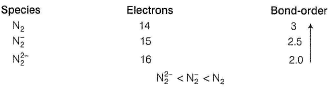Test: Molecular Orbital Theory - Question 8

O — F and F2 can be compared in terms of

Detailed Solution for Test: Molecular Orbital Theory - Question 8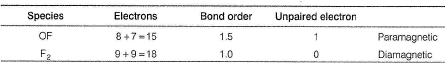Bond energy of O — F (1.5) > F — F (1)
Thus, OF is more stable than F2 thus, (c).

Test: Molecular Orbital Theory - Question 9

Which of the following diatomic molecules would be stabilised by the removed of an electron?

Detailed Solution for Test: Molecular Orbital Theory - Question 9

A molecule is stabilised if bond-order increases, an anti-bonding electron is lost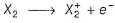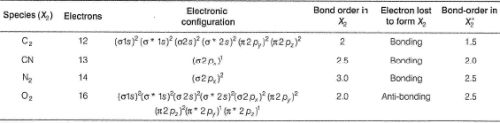Test: Molecular Orbital Theory - Question 10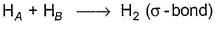Probability (electron charge density) of bonding and anti-bonding molecular orbitals are given.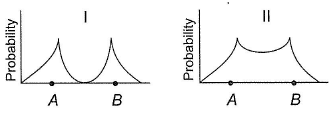Select the correct probability,

Detailed Solution for Test: Molecular Orbital Theory - Question 10

Electron-charge density in a bonding molecular orbital is high in the internuclear region as shown in II.
In an anti-bonding molecular orbital, it is high in parts of the molecule away from the internuclear region.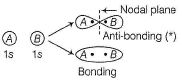Test: Molecular Orbital Theory - Question 11

If one of the electrons in the He2 molecule is taken to the next excited state, then bond order in He2

Detailed Solution for Test: Molecular Orbital Theory - Question 11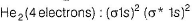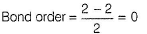Electron is taken to next excited state that is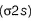hen electronic configuration is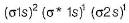Number of electrons in bonding molecular orbital = 3
and in anti-bonding molecular orbital = 1
Thus, bond order = (3 - 1) /2 =1
Thus, bond order increases by 1 unit.

Test: Molecular Orbital Theory - Question 12

If one of the electrons (1s2) of helium is taken in excited state then bond order of He2 is

Detailed Solution for Test: Molecular Orbital Theory - Question 12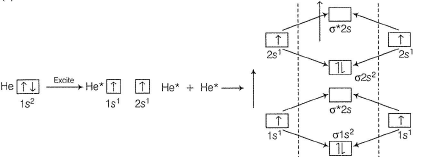Number of electrons in bonding orbital = 4 and in anti-bonding orbitai = 0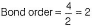Test: Molecular Orbital Theory - Question 13

The bond energy of H2 is 436 kJ mol -1. Thus, bond energy ofis

Detailed Solution for Test: Molecular Orbital Theory - Question 13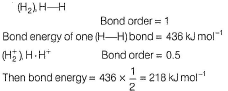Test: Molecular Orbital Theory - Question 14

Consider the following oxidation/reduction process,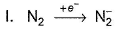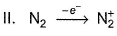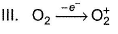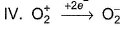Q. Magnetic moment does not change in

Detailed Solution for Test: Molecular Orbital Theory - Question 14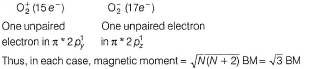Test: Molecular Orbital Theory - Question 15

Direction (Q. Nos. 15) This sectionis based on statement I and Statement II. Select the correct answer from the code given below.

Q.

Statement I : N2 has a greater dissociation energy than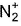, where as O2 has lower dissociation energy than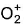.

Statement II : N2 has 14 electrons while O2 has 16 electrons .

Detailed Solution for Test: Molecular Orbital Theory - Question 15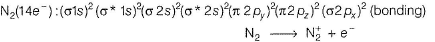Electron from bonding molecular orbital of higher stability is lost (requires higher energy).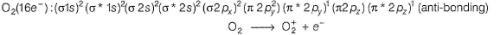Electron from antibonding molecular orbital of lower stability is lost (requires lower energy).
N2 has 14 electrons and O2 has 16 electrons.
Thus, both Statements I and II are correct but Statement II is not the correct explanation of Statement I

*Multiple options can be correct
Test: Molecular Orbital Theory - Question 16

Direction (Q. Nos. 16-18) This section contains 4 multiple choice questions. Each question has four choices (a), (b), (c) and (d), out of which ONE or  MORE THANT ONE  is correct.

Q. Set of species with identical bond order is/are

Detailed Solution for Test: Molecular Orbital Theory - Question 16

isoelectronic species will generally have identical bond order: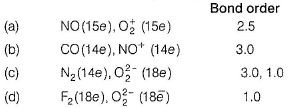*Multiple options can be correct
Test: Molecular Orbital Theory - Question 17

One of the electrons of the highest energy level is taken to next excited state in the following diatomic species. Select the species which undergoes change in bond order?

Detailed Solution for Test: Molecular Orbital Theory - Question 17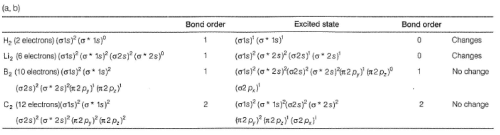*Multiple options can be correct
Test: Molecular Orbital Theory - Question 18

In which of the following processes, does the value of magnetic moment change ?

Detailed Solution for Test: Molecular Orbital Theory - Question 18

(a) CO (14e), no unpaired electron, magnetic moment = 0
CO+ (13e), One electron is lost from bonding melecular orbital unpaired electron = 1, magnetic moment =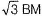(b)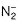(15e) Unpaired electron = 1, magnetic moment =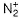(13e) Unpaired electron = 1, magnetic moment =(c) Zn, Zn2+, unpaired electron = 0
(d) O2 (16e), unpaired electron = 2, magnetic moment =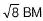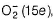, unpaired electron = 1, magnetic moment =Test: Molecular Orbital Theory - Question 19

Direction (Q. Nos. 19-20) This section contains  a paragraph, each describing theory, experiments, data etc. three Questions related to paragraph have been given.Each question have only one correct answer among the four given options (a),(b),(c),(d)

Valence shell MO electronic configuration of a diatomic species is shown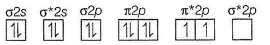* is for anti-bonding molecular orbital (MO).

Q. Bond order of this species is

Detailed Solution for Test: Molecular Orbital Theory - Question 19

Total number of electrons = 16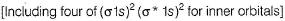Number of bonding electrons = 10,
Number of anti-bonding electrons = 6,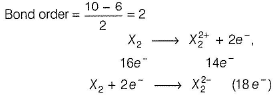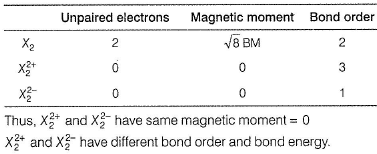Test: Molecular Orbital Theory - Question 20

Valence shell MO electronic configuration of a diatomic species is shown* is for anti-bonding molecular orbital (MO).

Q. Divalent cation of this species

Detailed Solution for Test: Molecular Orbital Theory - Question 20

Total number of electrons = 16Number of bonding electrons = 10, Number of anti-bonding electrons = 6,Test: Molecular Orbital Theory - Question 21

Direction (Q. Nos. 21) Choice the correct combination of elements and column I and coloumn II  are given as option (a), (b), (c) and (d), out of which ONE option is correct.

Q. Match the conversion in Column l with the type of effect given in Column II.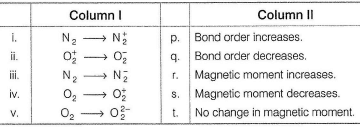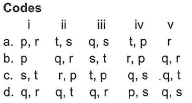Detailed Solution for Test: Molecular Orbital Theory - Question 21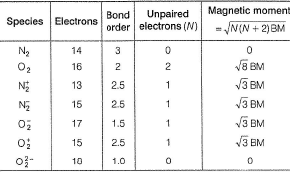Thus, (i) - (q , r)    (ii) - (q.t),
(iii) - (q,r),    (iv)- (P.s).
(v) - (q,s).

*Answer can only contain numeric values
Test: Molecular Orbital Theory - Question 22

Direction (Q. Nos. 22 and 23) This section contains 3 questions. when worked out will result in an integer from 0 to 9 (both inclusive).

Q. Total number of electrons in anti-bonding MO in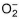(superoxide ion) is .......

Detailed Solution for Test: Molecular Orbital Theory - Question 22(17 electrons) has molecular orbital electronic configuration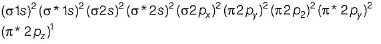Underlined are anti-bonding molecular orbital Thus, seven electrons are in anti-bonding molecular orbitals.

*Answer can only contain numeric values
Test: Molecular Orbital Theory - Question 23

How many bonding MO are used in the formation of NO?

Detailed Solution for Test: Molecular Orbital Theory - Question 23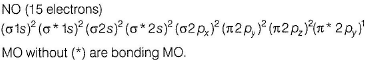## Topic-wise MCQ Tests for NEET

1493 tests
 Use Code STAYHOME200 and get INR 200 additional OFF Use Coupon Code
Information about Test: Molecular Orbital Theory Page
In this test you can find the Exam questions for Test: Molecular Orbital Theory solved & explained in the simplest way possible. Besides giving Questions and answers for Test: Molecular Orbital Theory, EduRev gives you an ample number of Online tests for practice

## Topic-wise MCQ Tests for NEET

1493 tests

### How to Prepare for NEET

Read our guide to prepare for NEET which is created by Toppers & the best Teachers